﻿ Gaps between Operator Norm and Spectral and Numerical Radii of the Tensor Product of OperatorsPublications are Open
Access in this journal
Article Versions
Export Article
• Normal Style
• MLA Style
• APA Style
• Chicago Style
Research Article
Open Access Peer-reviewed

### Gaps between Operator Norm and Spectral and Numerical Radii of the Tensor Product of Operators

Zameddin I. Ismailov , Pembe Ipek Al
Turkish Journal of Analysis and Number Theory. 2021, 9(2), 22-24. DOI: 10.12691/tjant-9-2-1
Received August 28, 2021; Revised October 02, 2021; Accepted October 11, 2021

### Abstract

One of the fundamental problem of the Spectral Theory of Linear Operators is to determine of the geometric place of the spectrum of the given operator and calculate the spectral and numerical radii of this operator. Other important problem in this theory is to explained the situation the spectral (numerical) radius is equal or not to operator norm. The only known way to calculate the spectral radius to date is the classical Gelfand formula, which often presents great technical challenges. Also, there is not yet a method of calculating the numerical radius for an operator. It should be noted that the finding the numerical range and numerical radius means maximally localizing the spectrum of an operator. The main purpose of this paper is to determine the relations gaps between operator norm and spectral and numerical radii of the tensor product operators associated with the compatible gaps of coordinate operators.

### 1. Introduction

As is known from the mathematical literature, in a Banach space one of the most fundamental questions of the Spectral Theory of Linear Operators is to clearly determine the geometric location of the spectrum or resolvent sets of the operator and calculate the spectral radius of the operator. Although in many cases, serious theoretical and technical difficulties are encountered in finding the spectrum set of nonselfadjoint linear operators, as it is known from the literature, the only formula used in the spectral radius of linear bounded operators calculation is Gelfand formula. Let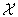be a Banach space and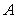be a linear bounded operator in, i.e.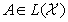, Gelfand formula is as follows: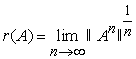1. As it is known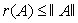. Letbe a linear bounded normal operator in a Hilbert space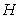. It is true the relation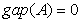(see 2). But generally, these equations may not be provided for non-normal linear bounded operators. For example, the gap between operator norm and spectral radius of the operator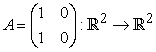is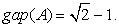Moreover, letbe a quasinilpotent operator. It is clear that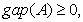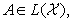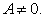The determination and definition the specific sub-classes of the operators family defined by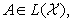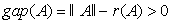is theoretically important as well as providing great benefits in applied mathematics.

Letbe a Hilbert space and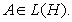It is known that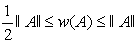3. If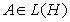is a normal operator, then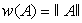(see 4). But this inequality may not be true for non-normal operators. Generally, forthe following inequality is true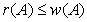(see 5, 6). One of the most fundamental questions is to identify specific subclasses of the family of operators that satisfy the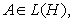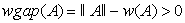condition.

In this study, the spectral and numerical gap characteristics of the tensor product of linear bounded operators defined on the tensor product of a finite number of Hilbert spaces are expressed with the appropriate characteristic numbers of the coordinate operators that form the tensor product.

Demuth's open problem in 2015 had a great impact on the emergence and shaping of the subject examined in this article (see 7).

### 2. Gap between Operator Norm and Spectral Radius of the Tensor Product of Operators

In this section, gap between operator norm and spectral radius of the tensor product of operator (for more information of tensor product see 8) will be investigated. It is known that for an operatorthe spectral radius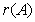is defined as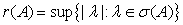(see 5). Namely, it is true the following result.

Theorem 1 Let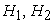be two separable Hilbert spaces and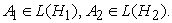For the tensor product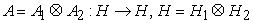is valid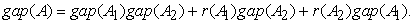Proof

By the definition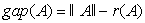it is known that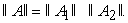From 9, for the spectrum ofit is known that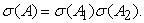Hence,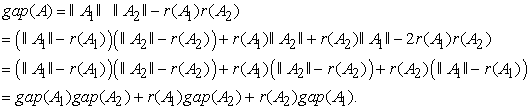Corollary 1 Under the assumptions of the last theorem it is true

1.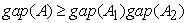,

2.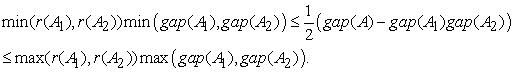Example 1 Let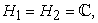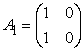and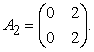In this case,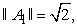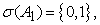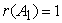and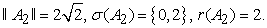Then,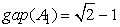and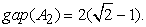Consequently, by Theorem 1 it is obtain that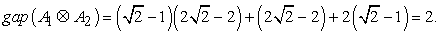Corollary 2 By using the last theorem for the tensor product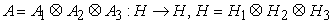of the operators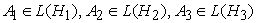is true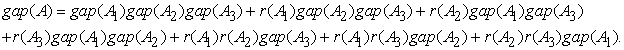Generally, using the Theorem 1 it can be proved the following proposition.

Corollary 3 For the tensor product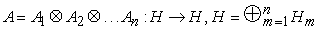of the operators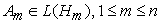it is true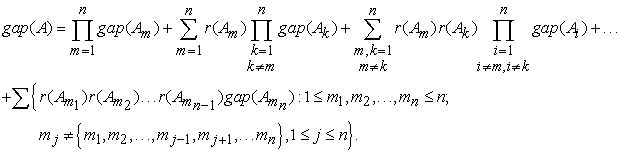Corollary 4 From the Corollary 3 we have

(1)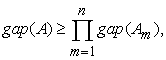(2)if and only if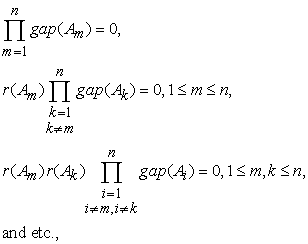(3) in special case when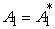and, then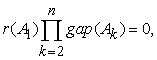(4) in special case when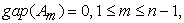then in order tothe necessary and sufficient condition is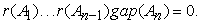### 3. Gap between Operator Norm and Numerical Radius of the Tensor Product of Operators

In this section, the gap between operator norm and numerical radius of the tensor product of operators associated with the gap of coordinate operators will be investigated.

Definition 1 3 The numerical range and the numerical radius of the linear bounded operator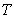in any Hilbert space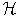define by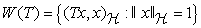and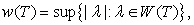respectively.

It is easy to prove the following proposition.

Theorem 2 For the numerical range and numerical radius of tensor product operator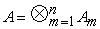of the operatorsin tensor productof Hilbert spaces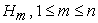are true.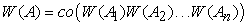and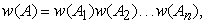where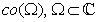denotes the convex hull of the set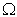.

Note that the analogous types result of Theorem 1 are true in this case too. For example, it is true the following result.

Theorem 3 For the tensor productof the operatorsin the tensor product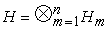of Hilbert spacesthe numerical gap is of the form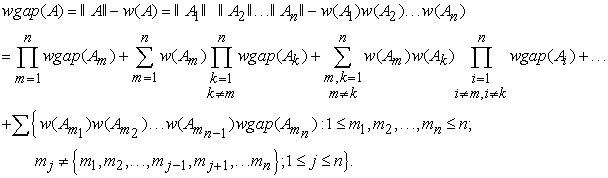Example 2 Let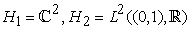and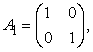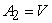Volterra integration operator in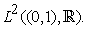In this case, we get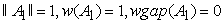and from 5 and 10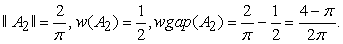Consequently, by Theorem 3 we have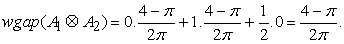### References

  I. M. Gelfand, Normierte ringe, Matematicheskii Sbornik, 9, 51 (1941), 3-24. In article  F. Hirsh, G. Lacombe, Elements of functional analysis, Springer, New York, 1999. In article  K. E. Gustafson, D. K. M. Rao, Numerical Range: The field of values of linear operators and matrices, Springer, New York, 1997. In article View Article  J. H. Anderson, C. Foias, Properties which normal operators share with normal derivations and related operators, Pacific Journal of Mathematics, 61, 2, 1975, 313-325. In article View Article  P. R. Halmos, A Hilbert space problem book, Van Nostrand, New York, 1967. In article  M. H. Stone, Linear transformations in Hilbert Space and their applications to analysis, Amer. Math. Soc. Colloquium Publications, 5, New York, 1932. In article View Article  PubMed  M. Demuth, Mathematical aspect of physics with non-selfadjoint operators, List of open problem, American Institute of Mathematics Workshop, 8-12 June 2015. In article  Y. M. Berezanskii, Z. G. Sheftel, G. F. Us, Functional analysis II, Birkhauser Verlag, Basel Switzerland, 1990. In article  A. Brown, C. Pearchy, Spectra of tensor products of operator, Proceedings of the American Mathematical Society 17, 1, 1966, 162-166. In article View Article  M. Gürdal, M. T. Garayev, S. Saltan, Some concrete operators and their properties, Turkish Journal of Mathematics, 39, 2015, 970-989. In article View Article

Published with license by Science and Education Publishing, Copyright © 2021 Zameddin I. Ismailov and Pembe Ipek AlThis work is licensed under a Creative Commons Attribution 4.0 International License. To view a copy of this license, visit http://creativecommons.org/licenses/by/4.0/